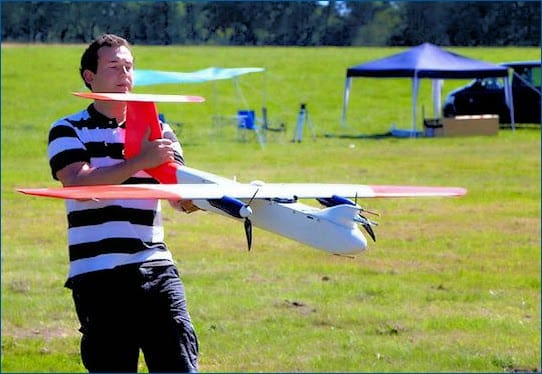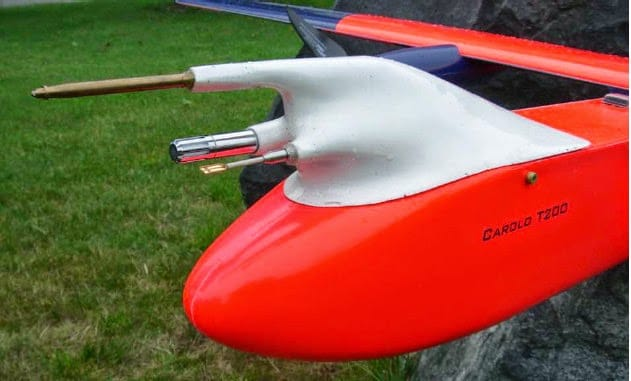Figure 1 – Meteorological UAV M2AV and meteorological measurement sensors. Article online here

Reliable measurement of the True Air Speed (TAS) of the aircraft is crucial for flights carried out by autopilot.  Knowing the TAS may be necessary to inline flight controller adaptation or reconfiguration. In this article, for example, airspeed is used as the switching parameter of a gain scheduling scheme.

To obtain an accurate TAS measurement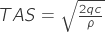it is necessary to calculate a precise value of the air density,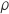. If our airframe is flying across the troposphere, the humidity modifies the air density.

We shall use for our density calculations the well-established CIPM Equation for the Determination of the Density of Moist Air (1981/91). Other density models, with a different range of applicability, can be chosen but this is not the main focus in this text.

Assuming an ISA atmosphere, the air density at 15°C and 101325 Pa is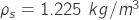, while the air is assumed to be dry. That value is by the CIPM equation. Moist air is a mix of dry air and water vapors. The molecular weight of dry air is about 28.96e-3 kg/mol, and the molecular weight of water vapor is 18e-3kg/mol. The higher the content of water vapor in the air, the lower the moist air density value is.

Let’s assume that the relative humidity value at ground level changes from 45% to 70%. In table 1 you find the air density value for different humidity values, dry air density is used to calculate the deviation shown in the last column of the table. For this calculations, you can use the density calculator script in the following link.

T=15°C, P=101325 Pa

RH %

Density kg/m3

Airspeed deviation %

0

1.225

0.00

45

1.222

0.12

70

1.22

0.20

The reported percentage airspeed variation expression is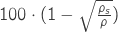and it is not dependent on the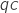value. The higher the operating temperature, the graver the effect of moist air is on airspeed readings: refer to the following table for numerical values at 35°C and 5°C.

T=15°C, P=101325 Pa

RH %

Density kg/m3

Airspeed deviation %

0

1.252

0.00

45

1.249

0.12

70

1.248

0.16

Extremal values, such as (0,70)RH and (5,35)°C, are plausible but should be carefully evaluated for each application. The precedent tables highlight that airspeed deviation increases with the operating temperature. Humidity is often not measured on DIY flying machines, but as shown here that can have an impact on the airspeed measurement accuracy. As usual, the overall accuracy of a measurement should be adapted to reflect the specific application. An additive uncertainty of 1% can be unacceptable for aerodynamic identification tasks but can be acceptable for less demanding flight control applications.